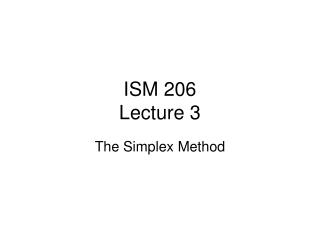Download PresentationISM 206 Lecture 3

# ISM 206 Lecture 3

Download Presentation## ISM 206 Lecture 3

- - - - - - - - - - - - - - - - - - - - - - - - - - - E N D - - - - - - - - - - - - - - - - - - - - - - - - - - -
##### Presentation Transcript

1. ISM 206Lecture 3 The Simplex Method

2. Announcements • Homework due 6pm Thursday • Thursday 6pm lecture

3. Outline • LP so far • Correction: Standard form • Why we can look only at basic feasible solutions • Optimality conditions • The simplex method • The step from one bfs to the next • Tableu method • Phase I: Finding an initial BFS

4. LP so far • Formulated LP’s in various contexts • Transform any LP into a standard form LP • Intuition of simplex method: Find the best corner point feasible solution • Math required: • Corner point Feasible or basic feasible solutions correspond to a set of n active constraints • Any set of active constraints corresponds to a basis from the matrix A • The basis is a set of linearly independent columns

5. Standard Form Concise version: A is an m by n matrix: n variables, m constraints

6. Solutions, Extreme points and bases • Key fact: • If a LP has an optimal solution, then it has an optimal extreme point solution (proved today) • Basic Feasible solution (Corner Point Feasible): • The vector x is an extreme point of the solution space iff it is a bfs of Ax=b, x>=0 • If A is of full rank then there is at least one basis B of A • B is set of linearly independent columns of A • B gives us a basic solution • If this is feasible then it is called a basic feasible solution (bfs) or corner point feasible (cpf)

7. Optimal basis theorem Theorem If a LP in standard form has a finite optimal solution then it has an optimal basic feasible solution Proof Requires the representation theorem…

8. Simplex Method • Checks the corner points • Gets better solution at each iteration 1. Find a starting solution 2. Test for optimality • If optimal then stop 3. Perform one iteration to new CPF (BFS) solution. Back to 2.

9. Simplex Method: basis change • One basic variable is replaced by another • The optimality test identifies a non-basic variable to enter the basis • The entering variable is increased until one of the other basic variables becomes zero • This is found using the minimum ratio test • That variable departs the basis

10. Standard Form to Augmented Form A is an m by n matrix: n variables, m constraints

11. The simplex method • Example • Table version

12. Questions and Break

13. A basic feasible solution • B=basis of A. • Write LP in terms of basis X is a basic solution of the LP X is a basic feasible solution if it is feasible! (example)

14. Optimality of a basis We want to test of a basic feasible solution is optimal Use the basic notation from before

15. Optimality test

16. Finding an initial bfs • The ‘phase 1’ approach • The ‘big M’ method

17. Proof that the Simplex method works • If there exists an optimal point, there exists an optimal basic feasible solution • There are a finite number of bfs • Each iteration, the simplex method moves from one bfs to another, and always improves the objective function value • Therefore the simplex method must converge to the optimal solution (in at most S steps, where S is the number of basic feasible solutions)